# class TSpectrum2Transform: public TObject

```THIS CLASS CONTAINS 2-DIMENSIONAL ORTHOGONAL TRANSFORM  FUNCTIONS.

These functions were written by:
Miroslav Morhac
Institute of Physics
Dubravska cesta 9, 842 28 BRATISLAVA
SLOVAKIA

email:fyzimiro@savba.sk,    fax:+421 7 54772479

The original code in C has been repackaged as a C++ class by R.Brun

The algorithms in this class have been published in the following
references:

 C.V. Hampton, B. Lian, Wm. C. McHarris: Fast-Fourier-transform
spectral enhancement techniques for gamma-ray spectroscopy.NIM A353
(1994) 280-284.
 Morhac M., Matousek V., New adaptive Cosine-Walsh  transform and
its application to nuclear data compression, IEEE Transactions on
Signal Processing 48 (2000) 2693.
 Morhac M., Matousek V., Data compression using new fast adaptive
Cosine-Haar transforms, Digital Signal Processing 8 (1998) 63.
 Morhac M., Matousek V.: Multidimensional nuclear data compression
using fast adaptive Walsh-Haar transform. Acta Physica Slovaca 51
(2001) 307.

```

## Function Members (Methods)

public:
 TSpectrum2Transform() TSpectrum2Transform(const TSpectrum2Transform&) TSpectrum2Transform(Int_t sizeX, Int_t sizeY) virtual ~TSpectrum2Transform() void TObject::AbstractMethod(const char* method) const virtual void TObject::AppendPad(Option_t* option = "") virtual void TObject::Browse(TBrowser* b) static TClass* Class() virtual const char* TObject::ClassName() const virtual void TObject::Clear(Option_t* = "") virtual TObject* TObject::Clone(const char* newname = "") const virtual Int_t TObject::Compare(const TObject* obj) const virtual void TObject::Copy(TObject& object) const virtual void TObject::Delete(Option_t* option = "")MENU virtual Int_t TObject::DistancetoPrimitive(Int_t px, Int_t py) virtual void TObject::Draw(Option_t* option = "") virtual void TObject::DrawClass() constMENU virtual TObject* TObject::DrawClone(Option_t* option = "") constMENU virtual void TObject::Dump() constMENU void Enhance(const Float_t** fSource, Float_t** fDest) virtual void TObject::Error(const char* method, const char* msgfmt) const virtual void TObject::Execute(const char* method, const char* params, Int_t* error = 0) virtual void TObject::Execute(TMethod* method, TObjArray* params, Int_t* error = 0) virtual void TObject::ExecuteEvent(Int_t event, Int_t px, Int_t py) virtual void TObject::Fatal(const char* method, const char* msgfmt) const void FilterZonal(const Float_t** fSource, Float_t** fDest) virtual TObject* TObject::FindObject(const char* name) const virtual TObject* TObject::FindObject(const TObject* obj) const virtual Option_t* TObject::GetDrawOption() const static Long_t TObject::GetDtorOnly() virtual const char* TObject::GetIconName() const virtual const char* TObject::GetName() const virtual char* TObject::GetObjectInfo(Int_t px, Int_t py) const static Bool_t TObject::GetObjectStat() virtual Option_t* TObject::GetOption() const virtual const char* TObject::GetTitle() const virtual UInt_t TObject::GetUniqueID() const virtual Bool_t TObject::HandleTimer(TTimer* timer) virtual ULong_t TObject::Hash() const virtual void TObject::Info(const char* method, const char* msgfmt) const virtual Bool_t TObject::InheritsFrom(const char* classname) const virtual Bool_t TObject::InheritsFrom(const TClass* cl) const virtual void TObject::Inspect() constMENU void TObject::InvertBit(UInt_t f) virtual TClass* IsA() const virtual Bool_t TObject::IsEqual(const TObject* obj) const virtual Bool_t TObject::IsFolder() const Bool_t TObject::IsOnHeap() const virtual Bool_t TObject::IsSortable() const Bool_t TObject::IsZombie() const virtual void TObject::ls(Option_t* option = "") const void TObject::MayNotUse(const char* method) const virtual Bool_t TObject::Notify() void TObject::Obsolete(const char* method, const char* asOfVers, const char* removedFromVers) const static void TObject::operator delete(void* ptr) static void TObject::operator delete(void* ptr, void* vp) static void TObject::operator delete[](void* ptr) static void TObject::operator delete[](void* ptr, void* vp) void* TObject::operator new(size_t sz) void* TObject::operator new(size_t sz, void* vp) void* TObject::operator new[](size_t sz) void* TObject::operator new[](size_t sz, void* vp) TSpectrum2Transform& operator=(const TSpectrum2Transform&) virtual void TObject::Paint(Option_t* option = "") virtual void TObject::Pop() virtual void TObject::Print(Option_t* option = "") const virtual Int_t TObject::Read(const char* name) virtual void TObject::RecursiveRemove(TObject* obj) void TObject::ResetBit(UInt_t f) virtual void TObject::SaveAs(const char* filename = "", Option_t* option = "") constMENU virtual void TObject::SavePrimitive(ostream& out, Option_t* option = "") void TObject::SetBit(UInt_t f) void TObject::SetBit(UInt_t f, Bool_t set) void SetDirection(Int_t direction) virtual void TObject::SetDrawOption(Option_t* option = "")MENU static void TObject::SetDtorOnly(void* obj) void SetEnhanceCoeff(Float_t enhanceCoeff) void SetFilterCoeff(Float_t filterCoeff) static void TObject::SetObjectStat(Bool_t stat) void SetRegion(Int_t xmin, Int_t xmax, Int_t ymin, Int_t ymax) void SetTransformType(Int_t transType, Int_t degree) virtual void TObject::SetUniqueID(UInt_t uid) virtual void ShowMembers(TMemberInspector&) virtual void Streamer(TBuffer&) void StreamerNVirtual(TBuffer& ClassDef_StreamerNVirtual_b) virtual void TObject::SysError(const char* method, const char* msgfmt) const Bool_t TObject::TestBit(UInt_t f) const Int_t TObject::TestBits(UInt_t f) const void Transform(const Float_t** fSource, Float_t** fDest) virtual void TObject::UseCurrentStyle() virtual void TObject::Warning(const char* method, const char* msgfmt) const virtual Int_t TObject::Write(const char* name = 0, Int_t option = 0, Int_t bufsize = 0) virtual Int_t TObject::Write(const char* name = 0, Int_t option = 0, Int_t bufsize = 0) const
protected:
 void BitReverse(Float_t* working_space, Int_t num) void BitReverseHaar(Float_t* working_space, Int_t shift, Int_t num, Int_t start) virtual void TObject::DoError(int level, const char* location, const char* fmt, va_list va) const void FourCos2(Float_t** working_matrix, Float_t* working_vector, Int_t numx, Int_t numy, Int_t direction, Int_t type) void Fourier(Float_t* working_space, Int_t num, Int_t hartley, Int_t direction, Int_t zt_clear) void General2(Float_t** working_matrix, Float_t* working_vector, Int_t numx, Int_t numy, Int_t direction, Int_t type, Int_t degree) Int_t GeneralExe(Float_t* working_space, Int_t zt_clear, Int_t num, Int_t degree, Int_t type) Int_t GeneralInv(Float_t* working_space, Int_t num, Int_t degree, Int_t type) void Haar(Float_t* working_space, Int_t num, Int_t direction) void HaarWalsh2(Float_t** working_matrix, Float_t* working_vector, Int_t numx, Int_t numy, Int_t direction, Int_t type) void TObject::MakeZombie() void Walsh(Float_t* working_space, Int_t num)

## Data Members

public:
 enum { kTransformHaar kTransformWalsh kTransformCos kTransformSin kTransformFourier kTransformHartley kTransformFourierWalsh kTransformFourierHaar kTransformWalshHaar kTransformCosWalsh kTransformCosHaar kTransformSinWalsh kTransformSinHaar kTransformForward kTransformInverse }; enum TObject::EStatusBits { kCanDelete kMustCleanup kObjInCanvas kIsReferenced kHasUUID kCannotPick kNoContextMenu kInvalidObject }; enum TObject::[unnamed] { kIsOnHeap kNotDeleted kZombie kBitMask kSingleKey kOverwrite kWriteDelete };
protected:
 Int_t fDegree degree of mixed transform, applies only for Fourier-Walsh, Fourier-Haar, Walsh-Haar, Cosine-Walsh, Cosine-Haar, Sine-Walsh, Sine-Haar transforms Int_t fDirection forward or inverse transform Float_t fEnhanceCoeff multiplication coefficient applied in enhanced region; Float_t fFilterCoeff value set in the filtered region Int_t fSizeX x length of transformed data Int_t fSizeY y length of transformed data Int_t fTransformType type of transformation (Haar, Walsh, Cosine, Sine, Fourier, Hartley, Fourier-Walsh, Fourier-Haar, Walsh-Haar, Cosine-Walsh, Cosine-Haar, Sine-Walsh, Sine-Haar) Int_t fXmax last channel x of filtered or enhanced region Int_t fXmin first channel x of filtered or enhanced region Int_t fYmax last channel y of filtered or enhanced region Int_t fYmin first channel y of filtered or enhanced region

## Class Charts## Function documentation

```default constructor
```
TSpectrum2Transform(Int_t sizeX, Int_t sizeY)
```the constructor creates TSpectrum2Transform object. Its sizes must be > than zero and must be power of 2.
It sets default transform type to be Cosine transform. Transform parameters can be changed using setter functions.
```

```destructor
```
void Haar(Float_t* working_space, Int_t num, Int_t direction)
```AUXILIARY FUNCION

This function calculates Haar transform of a part of data
Function parameters:
-working_space-pointer to vector of transformed data
-num-length of processed data
-direction-forward or inverse transform

```
void Walsh(Float_t* working_space, Int_t num)
```AUXILIARY FUNCION

This function calculates Walsh transform of a part of data
Function parameters:
-working_space-pointer to vector of transformed data
-num-length of processed data

```
void BitReverse(Float_t* working_space, Int_t num)
```AUXILIARY FUNCION

This function carries out bir-reverse reordering of data
Function parameters:
-working_space-pointer to vector of processed data
-num-length of processed data

```
void Fourier(Float_t* working_space, Int_t num, Int_t hartley, Int_t direction, Int_t zt_clear)
```AUXILIARY FUNCION

This function calculates Fourier based transform of a part of data
Function parameters:
-working_space-pointer to vector of transformed data
-num-length of processed data
-hartley-1 if it is Hartley transform, 0 othewise
-direction-forward or inverse transform

```
void BitReverseHaar(Float_t* working_space, Int_t shift, Int_t num, Int_t start)
```AUXILIARY FUNCION

This function carries out bir-reverse reordering for Haar transform
Function parameters:
-working_space-pointer to vector of processed data
-shift-shift of position of processing
-start-initial position of processed data
-num-length of processed data

```
Int_t GeneralExe(Float_t* working_space, Int_t zt_clear, Int_t num, Int_t degree, Int_t type)
```AUXILIARY FUNCION

This function calculates generalized (mixed) transforms of different degrees
Function parameters:
-working_space-pointer to vector of transformed data
-zt_clear-flag to clear imaginary data before staring
-num-length of processed data
-degree-degree of transform (see manual)
-type-type of mixed transform (see manual)

```
Int_t GeneralInv(Float_t* working_space, Int_t num, Int_t degree, Int_t type)
```AUXILIARY FUNCION

This function calculates inverse generalized (mixed) transforms
Function parameters:
-working_space-pointer to vector of transformed data
-num-length of processed data
-degree-degree of transform (see manual)
-type-type of mixed transform (see manual)

```
void HaarWalsh2(Float_t** working_matrix, Float_t* working_vector, Int_t numx, Int_t numy, Int_t direction, Int_t type)
```AUXILIARY FUNCION

This function calculates 2D Haar and Walsh transforms
Function parameters:
-working_matrix-pointer to matrix of transformed data
-working_vector-pointer to vector where the data are processed
-numx,numy-lengths of processed data
-direction-forward or inverse
-type-type of transform (see manual)

```
void FourCos2(Float_t** working_matrix, Float_t* working_vector, Int_t numx, Int_t numy, Int_t direction, Int_t type)
```AUXILIARY FUNCION

This function calculates 2D Fourier based transforms
Function parameters:
-working_matrix-pointer to matrix of transformed data
-working_vector-pointer to vector where the data are processed
-numx,numy-lengths of processed data
-direction-forward or inverse
-type-type of transform (see manual)

```
void General2(Float_t** working_matrix, Float_t* working_vector, Int_t numx, Int_t numy, Int_t direction, Int_t type, Int_t degree)
```AUXILIARY FUNCION

This function calculates generalized (mixed) 2D transforms
Function parameters:
-working_matrix-pointer to matrix of transformed data
-working_vector-pointer to vector where the data are processed
-numx,numy-lengths of processed data
-direction-forward or inverse
-type-type of transform (see manual)
-degree-degree of transform (see manual)

```
void Transform(const Float_t** fSource, Float_t** fDest)
```TWO-DIMENSIONAL TRANSFORM FUNCTION
This function transforms the source spectrum. The calling program
should fill in input parameters.
Transformed data are written into dest spectrum.

Function parameters:
fSource-pointer to the matrix of source spectrum, its size should
be fSizex*fSizey except for inverse FOURIER, FOUR-WALSH, FOUR-HAAR
transform. These need fSizex*2*fSizey length to supply real and
imaginary coefficients.
fDest-pointer to the matrix of destination data, its size should be
fSizex*fSizey except for direct FOURIER, FOUR-WALSh, FOUR-HAAR. These
need fSizex*2*fSizey length to store real and imaginary coefficients
fSizex,fSizey-basic dimensions of source and dest spectra

```

Transform methods

Goal: to analyze experimental data using orthogonal transforms

         orthogonal transforms can be successfully used for the processing of nuclear spectra (not only)

         they can be used to remove high frequency noise, to increase signal-to-background ratio as well as to enhance low intensity components , to carry out e.g. Fourier analysis etc.

         we have implemented the function for the calculation of the commonly used orthogonal transforms as well as functions for the filtration and enhancement of experimental data

Function:

void TSpectrumTransform2::Transform(const float **fSource, float **fDest)

This function transforms the source spectrum according to the given input parameters. Transformed data are written into dest spectrum. Before the Transform function is called the class must be created by constructor and the type of the transform as well as some other parameters must be set using a set of setter functions:

Member variables of TSpectrumTransform2 class:

fSource-pointer to the matrix of source spectrum. Its lengths should be equal to the fSizex, fSizey parameters except for inverse FOURIER, FOUR-WALSH, FOUR-HAAR transforms. These need 2*fSizex*fSizey length to supply real and imaginary coefficients.

fDest-pointer to the matrix of destination spectrum. Its lengths should be equal to the fSizex, fSizey parameters except for inverse FOURIER, FOUR-WALSH, FOUR-HAAR transforms. These need 2*fSizex*fSizey length to store real and imaginary coefficients.

fSizeX,fSizeY-basic lengths of the source and dest spectra. They should be power

of 2.

fType-type of transform

Classic transforms:

kTransformHaar

kTransformWalsh

kTransformCos

kTransformSin

kTransformFourier

kTransformHartley

Mixed transforms:

kTransformFourierWalsh

kTransformFourierHaar

kTransformWalshHaar

kTransformCosWalsh

kTransformCosHaar

kTransformSinWalsh

kTransformSinHaar

fDirection-direction-transform direction (forward, inverse)

kTransformForward

kTransformInverse

fDegree-applies only for mixed transforms , , .

Allowed range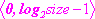.

References:

 C.V. Hampton, B. Lian, Wm. C. McHarris: Fast-Fourier-transform spectral enhancement techniques for gamma-ray spectroscopy. NIM A353 (1994) 280-284.

 Morháč M., Matouek V., New adaptive Cosine-Walsh  transform and its application to nuclear data compression, IEEE Transactions on Signal Processing 48 (2000) 2693.

 Morháč M., Matouek V., Data compression using new fast adaptive Cosine-Haar transforms, Digital Signal Processing 8 (1998) 63.

 Morháč M., Matouek V.: Multidimensional nuclear data compression using fast adaptive Walsh-Haar transform. Acta Physica Slovaca 51 (2001) 307.

Example 1  script Transform2.c: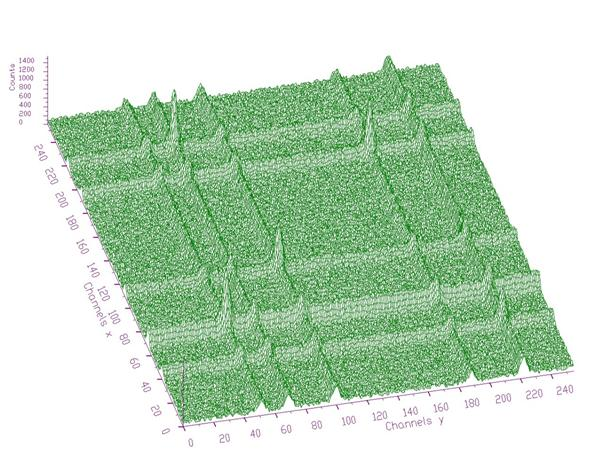Fig. 1 Original two-dimensional noisy spectrum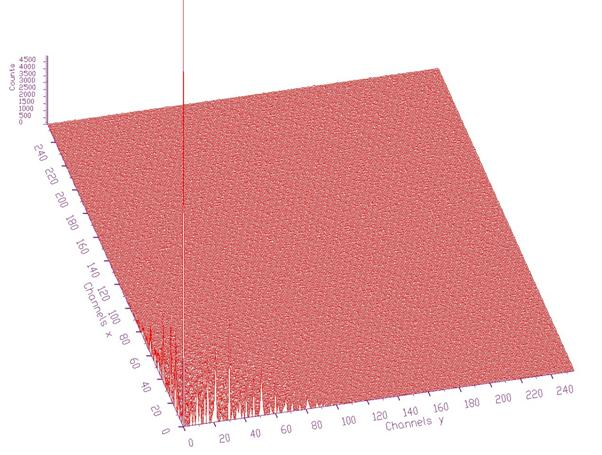Fig. 2 Transformed spectrum from Fig. 1 using Cosine transform. Energy of the trasnsformed data is concentrated around the beginning of the coordinate system

Script:

// Example to illustrate Transform function (class TSpectrumTransform2).

// To execute this example, do

// root > .x Transform2.C

void Transform2() {

Int_t i, j;

Int_t nbinsx = 256;

Int_t nbinsy = 256;

Int_t xmin  = 0;

Int_t xmax  = nbinsx;

Int_t ymin  = 0;

Int_t ymax  = nbinsy;

Float_t ** source = new float *[nbinsx];

Float_t ** dest = new float *[nbinsx];

for (i=0;i<nbinsx;i++)

source[i]=new float[nbinsy];

for (i=0;i<nbinsx;i++)

dest[i]=new float[nbinsy];

TH2F *trans = new TH2F("trans","Background estimation",nbinsx,xmin,xmax,nbinsy,ymin,ymax);

TFile *f = new TFile("TSpectrum2.root");

trans=(TH2F*) f->Get("back3;1");

TCanvas *Tr = new TCanvas("Transform","Illustation of transform function",10,10,1000,700);

for (i = 0; i < nbinsx; i++){

for (j = 0; j < nbinsy; j++){

source[i][j] = trans->GetBinContent(i + 1,j + 1);

}

}

TSpectrumTransform2 *t = new TSpectrumTransform2(256,256);

t->SetTransformType(t->kTransformCos,0);

t->SetDirection(t->kTransformForward);

t->Transform(source,dest);

for (i = 0; i < nbinsx; i++){

for (j = 0; j < nbinsy; j++){

trans->SetBinContent(i + 1, j + 1,dest[i][j]);

}

}

trans->Draw("SURF");

}

void FilterZonal(const Float_t** fSource, Float_t** fDest)
```TWO-DIMENSIONAL FILTER ZONAL FUNCTION
This function transforms the source spectrum. The calling program
should fill in input parameters. Then it sets transformed
coefficients in the given region to the given
filter_coeff and transforms it back
Filtered data are written into dest spectrum.

Function parameters:
fSource-pointer to the matrix of source spectrum, its size should
be fSizeX*fSizeY
fDest-pointer to the matrix of destination data, its size should be
fSizeX*fSizeY

```

Example of zonal filtering

Function:

void TSpectrumTransform2::FilterZonal(const float **fSource, float **fDest)

This function transforms the source spectrum (for details see Transform function).  Before the FilterZonal function is called the class must be created by constructor and the type of the transform as well as some other parameters must be set using a set of setter functions. The FilterZonal function sets transformed coefficients in the given region (fXmin, fXmax) to the given fFilterCoeff and transforms it back. Filtered data are written into dest spectrum.

Example   script Fitler2.c: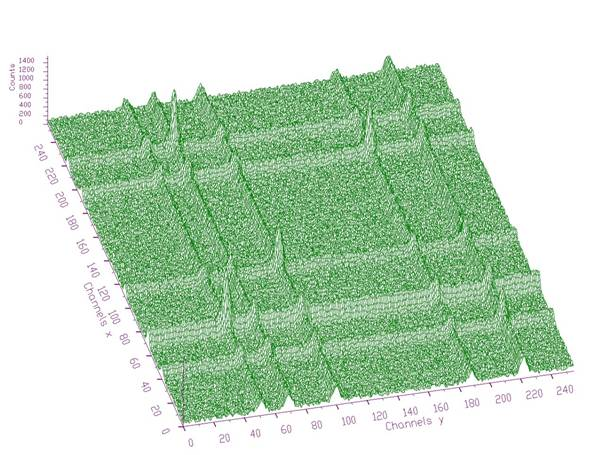Fig. 1 Original two-dimensional noisy spectrumFig. 2 Filtered spectrum using Cosine transform and zonal filtration (channels in regions (128-255)x(0-255) and (0-255)x(128-255) were set to 0).

Script:

// Example to illustrate zonal filtration (class TSpectrumTransform2).

// To execute this example, do

// root > .x Filter2.C

void Filter2() {

Int_t i, j;

Int_t nbinsx = 256;

Int_t nbinsy = 256;

Int_t xmin  = 0;

Int_t xmax  = nbinsx;

Int_t ymin  = 0;

Int_t ymax  = nbinsy;

Float_t ** source = new float *[nbinsx];

Float_t ** dest = new float *[nbinsx];

for (i=0;i<nbinsx;i++)

source[i]=new float[nbinsy];

for (i=0;i<nbinsx;i++)

dest[i]=new float[nbinsy];

TH2F *trans = new TH2F("trans","Background estimation",nbinsx,xmin,xmax,nbinsy,ymin,ymax);

TFile *f = new TFile("TSpectrum2.root");

trans=(TH2F*) f->Get("back3;1");

TCanvas *Tr = new TCanvas("Transform","Illustation of transform function",10,10,1000,700);

for (i = 0; i < nbinsx; i++){

for (j = 0; j < nbinsy; j++){

source[i][j] = trans->GetBinContent(i + 1,j + 1);

}

}

TSpectrumTransform2 *t = new TSpectrumTransform2(256,256);

t->SetTransformType(t->kTransformCos,0);

t->SetRegion(0,255,128,255);

t->FilterZonal(source,dest);

for (i = 0; i < nbinsx; i++){

for (j = 0; j < nbinsy; j++){

source[i][j] = dest[i][j];

}

}

t->SetRegion(128,255,0,255);

t->FilterZonal(source,dest);

trans->Draw("SURF");

}

void Enhance(const Float_t** fSource, Float_t** fDest)
```TWO-DIMENSIONAL ENHANCE ZONAL FUNCTION
This function transforms the source spectrum. The calling program
should fill in input parameters. Then it multiplies transformed
coefficients in the given region by the given
enhance_coeff and transforms it back

Function parameters:
fSource-pointer to the matrix of source spectrum, its size should
be fSizeX*fSizeY
fDest-pointer to the matrix of destination data, its size should be
fSizeX*fSizeY

```

Example of enhancement

Function:

void TSpectrumTransform2::Enhance(const float **fSource, float **fDest)

This function transforms the source spectrum (for details see Transform function).  Before the Enhance function is called the class must be created by constructor and the type of the transform as well as some other parameters must be set using a set of setter functions. The Enhance function multiplies transformed coefficients in the given region (fXmin, fXmax, fYmin, fYmax) by the given fEnhancCoeff and transforms it back. Enhanced data are written into dest spectrum.

Example  script Enhance2.c:Fig. 1 Original two-dimensional noisy spectrum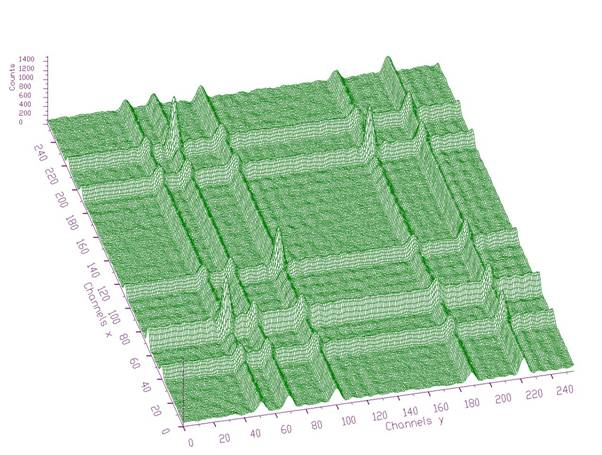Fig. 2 Enhanced spectrum of the data from Fig. 1 using Cosine transform (channels in region (0-63)x(0-63) were multiplied by 5)

Script:

// Example to illustrate enhancement (class TSpectrumTransform2).

// To execute this example, do

// root > .x Enhance2.C

void Enhance2() {

Int_t i, j;

Int_t nbinsx = 256;

Int_t nbinsy = 256;

Int_t xmin  = 0;

Int_t xmax  = nbinsx;

Int_t ymin  = 0;

Int_t ymax  = nbinsy;

Float_t ** source = new float *[nbinsx];

Float_t ** dest = new float *[nbinsx];

for (i=0;i<nbinsx;i++)

source[i]=new float[nbinsy];

for (i=0;i<nbinsx;i++)

dest[i]=new float[nbinsy];

TH2F *trans = new TH2F("trans","Background estimation",nbinsx,xmin,xmax,nbinsy,ymin,ymax);

TFile *f = new TFile("TSpectrum2.root");

trans=(TH2F*) f->Get("back3;1");

TCanvas *Tr = new TCanvas("Transform","Illustation of transform function",10,10,1000,700);

for (i = 0; i < nbinsx; i++){

for (j = 0; j < nbinsy; j++){

source[i][j] = trans->GetBinContent(i + 1,j + 1);

}

}

TSpectrumTransform2 *t = new TSpectrumTransform2(256,256);

t->SetTransformType(t->kTransformCos,0);

t->SetRegion(0,63,0,63);

t->SetEnhanceCoeff(5);

t->Enhance(source,dest);

trans->Draw("SURF");

}

void SetTransformType(Int_t transType, Int_t degree)
```   SETTER FUNCION

This function sets the following parameters for transform:
-transType - type of transform (Haar, Walsh, Cosine, Sine, Fourier, Hartley, Fourier-Walsh, Fourier-Haar, Walsh-Haar, Cosine-Walsh, Cosine-Haar, Sine-Walsh, Sine-Haar)
-degree - degree of mixed transform, applies only for Fourier-Walsh, Fourier-Haar, Walsh-Haar, Cosine-Walsh, Cosine-Haar, Sine-Walsh, Sine-Haar transforms

```
void SetRegion(Int_t xmin, Int_t xmax, Int_t ymin, Int_t ymax)
```   SETTER FUNCION

This function sets the filtering or enhancement region:
-xmin, xmax, ymin, ymax

```
void SetDirection(Int_t direction)
```   SETTER FUNCION

This function sets the direction of the transform:
-direction (forward or inverse)

```
void SetFilterCoeff(Float_t filterCoeff)
```   SETTER FUNCION

This function sets the filter coefficient:
-filterCoeff - after the transform the filtered region (xmin, xmax, ymin, ymax) is replaced by this coefficient. Applies only for filtereng operation.

```
void SetEnhanceCoeff(Float_t enhanceCoeff)
```   SETTER FUNCION

This function sets the enhancement coefficient:
-enhanceCoeff - after the transform the enhanced region (xmin, xmax, ymin, ymax) is multiplied by this coefficient. Applies only for enhancement operation.

```